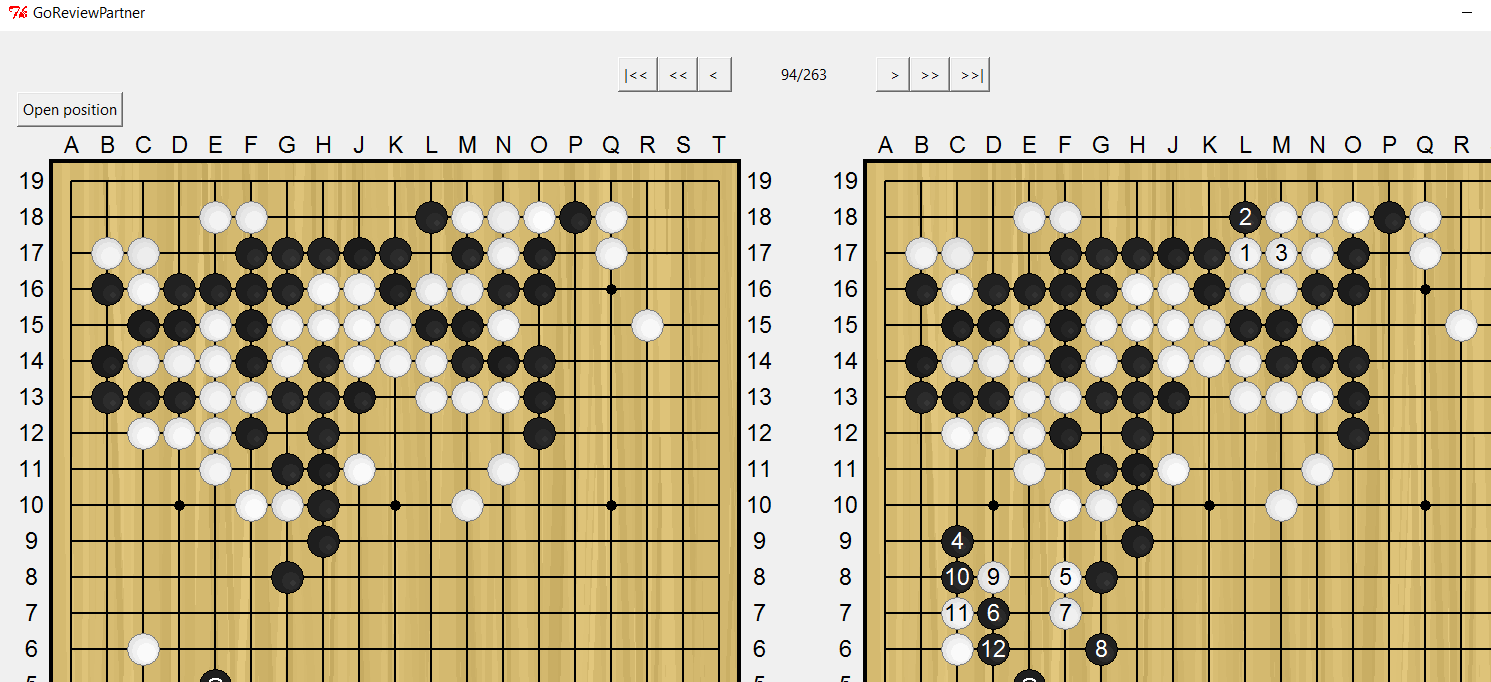# pnprog/goreviewpartner

Have a question about this project? Sign up for a free GitHub account to open an issue and contact its maintainers and the community.

By clicking “Sign up for GitHub”, you agree to our terms of service and privacy statement. We’ll occasionally send you account related emails.

# Sometimes in some variations, a player plays on the position where a stone is already there. #29

Closed
opened this Issue Mar 8, 2018 · 7 comments

Projects
None yet

### liverex commented Mar 8, 2018

 Please try the attached rsgf file which I generated. At Variation A of step 94/263, black 2 is at L18 where a black stone is already over there. I'm using the latest code.Author

### liverex commented Mar 8, 2018 • edited

 (;FFAP[Sabaki:0.33.1]BOT[Leela Zero]BOTV[0.12]BR[18级]CA[UTF-8] DT[2018-03-08]GMKM[6.5]PB[zip12345]PW[moya11]RE[B+0] RSGF[Analysis by GoReviewPartner Bot: Leela Zero/0.12 Komi: 6.5 Intervals: 90-100 (both colors) ] SZWR[18级];B[pp];W[cd];B[dp];W[pc];B[jp];W[qe];B[ed];W[hd];B[dg];W[ee]; B[fe];W[ef];B[dd];W[cf];B[de];W[df];B[ce];W[cc];B[bd];W[eb];B[fc];W[fb];B[ff]; W[eg];B[bf];W[fg];B[gg];W[gh];B[gc];W[bc];B[hh];W[gf];B[hg];W[ge];B[fd];W[ch]; B[cg];W[dh];B[bg];W[ei];B[gi];W[cn];B[fh];W[eh];B[hc];W[id];B[ic];W[kd];B[jd]; W[je];B[jc];W[if];B[ke];W[kf];B[le];W[ld];B[md];W[me];B[lf];W[mc];B[nd];W[lg]; B[mf];W[mg];B[nf];W[lb];B[nc];W[nb];B[ob];W[kg];B[hf];W[he];B[gd];W[ie];B[ig]; W[jf];B[ng];W[mi];B[nh];W[lj];B[gl];W[fj];B[hk];W[gj];B[hj];W[ii];B[hi];W[pb]; B[lc](;CBM[L17]ES[W+6.5]VNWR[97.74%/2.26%]W[mb](;B[kb]CBM[L18]ES[W+3.5] VNWR[99.10%/0.9%];CBM[resign]ES[W+3.5]W[fq](;B[eo]CBM[P17]ES[W+4.5] VNWR[92.98%/7.02%](;CBM[L17]ES[W+7.5]VNWR[83.65%/16.35%]W[cq](;B[cp]CBM[D3] ES[W+4.5]VNWR[91.06%/8.94%](;CBM[C9]ES[W+5.5]VNWR[84.6%/15.40%]W[dq](;B[dl] CBM[C8]ES[W+4.5]VNWR[90.60%/9.4%](;CBM[C9]ES[W+5.5]VNWR[87.42%/12.58%]W[fl](; B[fm]CBM[F7]ES[W+4.5]VNWR[90.42%/9.58%](;CBM[C9]ES[W+5.5]VNWR[88.58%/11.42%] W[el];B[em];W[dk];B[ck];W[cl];B[dm];W[cj];B[bk];W[bj];B[ek];W[dj];B[hq];W[ep]; B[do];W[qq];B[pq];W[qp];B[po];W[qo];B[qn];W[rn];B[nj];W[ni];B[oi];W[nk];B[mh]; W[lh];B[mj];W[li];B[lk];W[mk];B[oj];W[kk];B[ll];W[kl];B[km];W[lm];B[ml];W[mm]; B[nl];W[jm];B[kn];W[il];B[fk];W[jn];B[ko];W[jo];B[kp];W[ip];B[iq];W[hp];B[jj]; W[ij];B[ik];W[kj];B[jk];W[jl];B[hn];W[gq];B[io];W[ok];B[ol];W[hl];B[hm];W[gk]; B[fi];W[ej];B[ji];W[pk];B[pl];W[qm];B[qk];W[ql];B[pj];W[pn];B[on];W[pr];B[or]; W[qr];B[nq];W[ho];B[in];W[hr];B[ir];W[gr];B[fp];W[gp];B[eq];W[er];B[dc];W[ad]; B[be];W[db];B[cm];W[bl];B[bm];W[ak];B[bq];W[br];B[bp];W[ds];B[qg];W[rf];B[oc]; W[oa];B[pd];W[qd];B[na];W[ma];B[ka];W[na];B[rg];W[pa];B[qf];W[se];B[pe];W[fo]; B[ep];W[gn];B[fn];W[go];B[gm];W[is];B[jr];W[js];B[ks];W[hs];B[kr];W[aq];B[ar]; W[bs];B[ap];W[rk];B[rj];W[rl];B[sj];W[gb];B[ae];W[ac];B[hb];W[ha];B[ia];W[ga]; B[bh];W[bi];B[pm];W[am];B[an];W[al];B[sk];W[sl];B[qn];W[sn];B[rc];W[rd];B[qc]; W[rb];B[sg];W[sf];B[sb];W[qb];B[sc];W[ra];B[la])(;PLYOPNV[33.52%] VNWR[88.58%/11.42%]W[ck];B[cl];W[bl];B[bm];W[bk];B[bn];W[el];B[dk];W[dj];B[ek]; W[bh];B[kb];W[qq];B[pq];W[qp])(;PLYOPNV[56.26%]VNWR[90.13%/9.87%]W[el]; B[em];W[dk];B[ck];W[cl];B[dm];W[bk];B[cj];W[dj];B[bj];W[ci];B[bl];W[kc];B[kb]; W[lc];B[oc];W[oa];B[na];W[ma];B[ka])(;PLYOPNV[3.49%]VNWR[92.12%/7.88%]W[cl] ;B[ck];W[dk];B[bl];W[cm];B[el];W[bk];B[cj])(;PLYOPNV[2.81%] VNWR[94.45%/5.55%]W[kc];B[kb];W[lc];B[ck];W[bh];B[bj])(;PLYOPNV[1.28%] VNWR[92.97%/7.03%]W[ad];B[be];W[ck];B[cl];W[bl];B[bm]))(;B[fm]PLYO PNV[70.47%]VNWR[90.42%/9.58%];W[ck];B[cl];W[bl];B[bm];W[bk];B[bn];W[el];B[dk]; W[dj];B[ek];W[bh];B[kb];W[oj];B[mk])(;B[dj]PLYOPNV[12.77%] VNWR[89.36%/10.64%];W[fm];B[dn];W[gn];B[gp];W[in];B[jl];W[km])(;B[ck]PLYO PNV[7.53%]VNWR[89.34%/10.66%];W[fm];B[dn];W[gm];B[hm];W[hn];B[im];W[go];B[in]; W[kc];B[kb])(;B[mh]PLYOPNV[1.36%]VNWR[90.65%/9.35%];W[lh];B[fm];W[ck];B[cl] )(;B[ek]PLYOPNV[1.59%]VNWR[90.28%/9.72%];W[fk];B[fm];W[ck];B[cl];W[bl]; B[bm];W[bk];B[bn])(;B[em]PLYOPNV[2.34%]VNWR[86.99%/13.01%];W[ck];B[cl]; W[bl];B[bm];W[bk]))(;PLYOPNV[47.50%]VNWR[87.42%/12.58%]W[ck];B[cl];W[bl]; B[bm];W[bk];B[bn];W[bh];B[kb];W[oj];B[ek];W[dk];B[mk];W[ni])(;PLYO PNV[10.80%]VNWR[91.99%/8.01%]W[cl];B[ck];W[dk];B[cj];W[dj];B[bl];W[cm];B[el])(; PLYOPNV[9.35%]VNWR[94.08%/5.92%]W[el];B[dm];W[ck];B[bl];W[dk];B[em])(; PLYOPNV[8.56%]VNWR[94.84%/5.16%]W[bk];B[ck];W[bl];B[cj];W[bj])(;PLYO PNV[6.71%]VNWR[94.24%/5.76%]W[kc];B[ck];W[oj];B[pf];W[oe];B[pe])(;PLYO PNV[5.74%]VNWR[93.43%/6.57%]W[fl];B[fm];W[el];B[em];W[dk];B[ck];W[cl];B[dm]; W[bk])(;PLYOPNV[2.65%]VNWR[89.88%/10.12%]W[ad];B[be];W[ck];B[cl];W[bl]; B[bm];W[bk];B[bn];W[bh];B[kb])(;PLYOPNV[3.65%]VNWR[92.79%/7.21%]W[dk];B[cl] ;W[ck];B[bl];W[el]))(;B[cl]PLYOPNV[19.49%]VNWR[90.60%/9.4%];W[dl];B[dm]; W[ck];B[cm];W[bk];B[el];W[dk];B[bh])(;B[ck]PLYOPNV[35.28%] VNWR[89.61%/10.39%];W[dl];B[cl];W[dm];B[dj];W[fl];B[fm];W[ek];B[bh];W[ci];B[bi] ;W[cj];B[bj])(;B[dl]PLYOPNV[14.31%]VNWR[89.86%/10.14%];W[ck];B[cl];W[bl]; B[bm];W[bk];B[bn];W[bh];B[ek];W[dk];B[oj];W[ml];B[ol];W[km])(;B[dk]PLYO PNV[4.23%]VNWR[90.58%/9.42%];W[fl];B[dm];W[fm];B[gn];W[bk];B[bl];W[cl];B[ck])(; B[bq]PLYOPNV[8.83%]VNWR[87.73%/12.27%];W[br];B[ck];W[dl];B[cl];W[dm];B[dj]; W[fl])(;B[oc]PLYOPNV[4.29%]VNWR[89.46%/10.54%];W[oa];B[ck];W[dl];B[cl]; W[dm];B[dj];W[fl];B[fm])(;B[mh]PLYOPNV[3.09%]VNWR[88.88%/11.12%];W[lh]; B[ki];W[li];B[oj];W[kc];B[kb];W[lc];B[ck])(;B[oj]PLYOPNV[2.20%] VNWR[88.20%/11.8%];W[dl];B[gp];W[ll];B[gq])(;B[mk]PLYOPNV[1.50%] VNWR[86.08%/13.92%];W[lk];B[ml];W[ll];B[lm])(;B[nj]PLYOPNV[1.32%] VNWR[85.78%/14.22%];W[ll];B[nl];W[dl];B[gp];W[kc]))(;PLYOPNV[2.74%] VNWR[84.6%/15.40%]W[ck];B[oc];W[oa];B[kb];W[dq];B[gp];W[gq];B[hp];W[oj];B[mk]; W[ni];B[pl])(;PLYOPNV[79.56%]VNWR[90.63%/9.37%]W[dq];B[ck];W[dl];B[cl]; W[dm];B[dj];W[fl];B[fm];W[ek];B[bh];W[fn];B[en])(;PLYOPNV[6.23%] VNWR[86.37%/13.63%]W[dl];B[dq];W[kc];B[kb];W[lc];B[bh];W[bi];B[bj];W[ci];B[ai]; W[ck];B[bl])(;PLYOPNV[5.18%]VNWR[87.02%/12.98%]W[kc];B[kb];W[lc];B[ck]; W[dl];B[cl];W[dm];B[bh];W[fm]))(;B[dq]PLYOPNV[53.21%]VNWR[91.06%/8.94%]; W[cp];B[dl];W[ck];B[cl];W[bl];B[bm];W[bk];B[cm];W[kc];B[kb];W[lc];B[bi];W[bh]; B[ah])(;B[cl]PLYOPNV[3.94%]VNWR[93.27%/6.73%];W[dl];B[dk];W[ek];B[dm]; W[el];B[cm];W[ck];B[bk];W[dj];B[dq])(;B[dl]PLYOPNV[8.87%]VNWR[90.60%/9.4%]; W[ck];B[cl];W[bl];B[bm];W[bk];B[dq];W[cp];B[cm];W[bn];B[dn];W[am])(;B[ck] PLYOPNV[8.21%]VNWR[90.54%/9.46%];W[dl];B[dj];W[fl];B[fm];W[el];B[bh];W[ci]) (;B[mh]PLYOPNV[3.05%]VNWR[92.54%/7.46%];W[lh];B[dq];W[cp];B[dm];W[cm];B[dl] ;W[ck];B[nj])(;B[er]PLYOPNV[4.08%]VNWR[91.39%/8.61%];W[cp];B[dm];W[cl]; B[cm];W[bm];B[bn];W[bo])(;B[cp]PLYOPNV[2.92%]VNWR[92.02%/7.98%];W[dq];B[ck] ;W[dl];B[cl];W[dm];B[dj];W[fl];B[fm];W[ek])(;B[oc]PLYOPNV[6.08%] VNWR[89.92%/10.08%];W[oa];B[dq];W[cp];B[dm];W[kc];B[kb];W[lc])(;B[oj]PLYO PNV[2.67%]VNWR[91.99%/8.01%];W[ll];B[dq];W[cp];B[dm];W[ck];B[cm];W[dr])(;B[mk] PLYOPNV[1.14%]VNWR[89.86%/10.14%];W[lk];B[ml];W[ll];B[lm])(;B[nj]PLYO PNV[1.30%]VNWR[89.10%/10.9%];W[ll];B[dq];W[cp]))(;PLYOPNV[9.68%] VNWR[83.65%/16.35%]W[kc];B[kb];W[lc];B[ck];W[dl];B[bh];W[dk];B[cj];W[fn];B[dj]; W[fl])(;PLYOPNV[47.61%]VNWR[86.34%/13.66%]W[dl];B[oc];W[oa];B[kb];W[oj]; B[mk];W[ni];B[kl];W[pl])(;PLYOPNV[5.84%]VNWR[88.01%/11.99%]W[ck];B[dm]; W[cm];B[dl];W[cl];B[bh];W[bi])(;PLYOPNV[6.50%]VNWR[89.82%/10.18%]W[em]; B[oc];W[oa];B[kb];W[fn];B[gp];W[hn])(;PLYOPNV[10.27%]VNWR[94.43%/5.57%] W[cq];B[dq];W[cp];B[dm];W[cm];B[dl];W[bk];B[oj];W[ll])(;PLYOPNV[6.52%] VNWR[91.58%/8.42%]W[fm];B[fl];W[dl];B[em];W[el];B[dm])(;PLYOPNV[3.03%] VNWR[89.9%/10.10%]W[cl];B[bh];W[bi];B[bj];W[ci];B[ai])(;PLYOPNV[1.73%] VNWR[92.78%/7.22%]W[el];B[dm];W[cl];B[dl])(;PLYOPNV[1.49%]VNWR[94.52%/5.48%] W[ad];B[be]))(;B[oc]PLYOPNV[21.04%]VNWR[92.98%/7.02%];W[oa];B[dl];W[cl]; B[ck];W[dk];B[bj];W[el];B[dm];W[em])(;B[kb]PLYOPNV[31.69%] VNWR[89.41%/10.59%];W[eo];B[dl];W[ck];B[cl];W[bl];B[bm];W[bk];B[cm];W[bn];B[an] ;W[ao];B[am];W[bp])(;B[dl]PLYOPNV[10.95%]VNWR[92.74%/7.26%];W[ck];B[cl]; W[bl];B[bm];W[bk];B[cm];W[kc];B[kb];W[lc];B[bh];W[bi];B[ek];W[dj];B[ci];W[ai])( ;B[mh]PLYOPNV[4.99%]VNWR[93.11%/6.89%];W[lh];B[ki];W[li];B[oj];W[kc];B[kb] ;W[lc];B[ll];W[ml])(;B[cl]PLYOPNV[7.50%]VNWR[92.52%/7.48%];W[dl];B[dm]; W[cm];B[ck];W[dk];B[dj];W[ek])(;B[dm]PLYOPNV[4.52%]VNWR[92.48%/7.52%];W[cl] ;B[cm];W[bm];B[dn];W[co];B[do];W[bk];B[cp];W[iq])(;B[fp]PLYOPNV[4.57%] VNWR[87.54%/12.46%];W[gp];B[fo];W[kc];B[kb];W[lc];B[ck];W[dl];B[bh])(;B[eq] PLYOPNV[3.39%]VNWR[88.16%/11.84%];W[dl];B[oc];W[oa];B[kb])(;B[oj]PLYO PNV[1.04%]VNWR[92.17%/7.83%];W[eo];B[dl];W[ck];B[cl];W[bl])(;B[eo]PLYO PNV[1.49%]VNWR[90.69%/9.31%];W[dl];B[oc];W[oa];B[kb];W[oj];B[mk];W[ni])(;B[ck] PLYOPNV[1.35%]VNWR[90.03%/9.97%];W[dl];B[cl];W[dm];B[bh]))(;B[kb]PLYO PNV[74.26%]VNWR[99.10%/0.9%];W[dl];B[oc];W[oa];B[dn];W[dm];B[co];W[en])(;B[oc] PLYOPNV[9.02%]VNWR[99.16%/0.84%];W[oa];B[kb];W[dl];B[na];W[ma];B[nj];W[ll]; B[nl])(;B[dl]PLYOPNV[3.65%]VNWR[99.59%/0.41%];W[kc];B[kb];W[lc];B[ck];W[oj] ;B[qg];W[qi];B[pe])(;B[mh]PLYOPNV[2.39%]VNWR[98.66%/1.34%];W[lh];B[ki]; W[li];B[oj];W[kc];B[kb];W[lc])(;B[cl]PLYOPNV[2.13%]VNWR[98.79%/1.21%];W[dl] ;B[dm];W[cm];B[ck];W[dk])(;B[ck]PLYOPNV[1.52%]VNWR[98.50%/1.5%];W[dl];B[dj] ;W[fl];B[fm])(;B[kc]PLYOPNV[1.64%]VNWR[98.24%/1.76%];W[dl];B[oc];W[oa]; B[ll];W[oj];B[mk])(;B[oj]PLYOPNV[1.18%]VNWR[98.88%/1.12%];W[kc];B[kb];W[lc] ))(;PLYOPNV[83.22%]VNWR[97.74%/2.26%]W[kc];B[kb];W[dl];B[bh];W[bi];B[bj]; W[ci];B[ai];W[ad];B[be];W[ck];B[co])(;PLYOPNV[3.48%]VNWR[98.01%/1.99%]W[em] ;B[mb];W[kl];B[co];W[bn];B[fn])(;PLYOPNV[3.30%]VNWR[98.73%/1.27%]W[dl]; B[mb];W[kl];B[ki];W[li])(;PLYOPNV[2.09%]VNWR[98.49%/1.51%]W[el];B[dm];W[kc] ;B[kb];W[cl])(;PLYOPNV[1.51%]VNWR[99.19%/0.81%]W[fl];B[fm];W[el];B[dn];W[dm] )(;PLYOPNV[1.41%]VNWR[99.31%/0.69%]W[oj];B[mb];W[dl];B[mk]))
Owner

### pnprog commented Mar 8, 2018

 Hi! Thanks for reporting this issue, I just pushed a modification that should fix that. In fact, yesterday, I post on L19 a message that was describing a strange behaviour by Leela and Leela Zero (full message there): I also noticed some behaviour that may be a violation of the GTP protocol for Leela and Leela Zero: When they choose to resign, they create a move in their move history that requires to be undo if one want to go back in the game tree. GnuGo does not consider "Resign" as a valid move. GoGui also agrees with GnuGo interpretation of the standard. Sabaki avoid this issue by replacing "undo" by "clearboard" and replaying the whole game up to the previous move. Ray does not undo. AQ undo command is broken at the moment (did not check for the previous version behaviour). I think this is an area where the GTP protocol documentation is not clear enough. I did not opened an issue for this one, seems everybody has its work around. But clearly, this poses problem when using Leela/LeelaZero with Gogui. Apparently this has been fixed since v0.9 (the version I was using). In your case, during the analysis, Leela Zero would have resign after black 91 at L18. Then when undoing Leela's answer to black 91 at L18 (so white 92 resign) I was in fact undoing black 91 because the new version of Leela Zero does not requires to undo a resign move. The whole analysis following 91 is wrong because Leela Zero believe there is no stone at L18. Let me know if this problem happens again.
Author

Author

Owner

### pnprog commented Mar 9, 2018

 My guess is that Leela Zero want to resign as white for every moves from 92 to 100. Does the RSGF file says something like this in the lest side comment box? Move XXX White to play, in the game, white played YYY For this position, Leela Zero would play: resign I plan to modify the code so that the variations are available even if Leela Zero wants to resign. In the mean time, I seem to remember there is a command line parameter for Leela Zero to change the resig threshold, maybe it's -r ?
Owner

### pnprog commented Mar 9, 2018

 Hi! I just went ahead and modified the code so that the variations are saved even when Leela/LeelaZero wants to resign. Please have a try and let me know :)
Author

### liverex commented Mar 12, 2018

 Thank you very much for your fix.

###liverex closed this Mar 25, 2018

to join this conversation on GitHub. Already have an account? Sign in to comment
You can’t perform that action at this time.